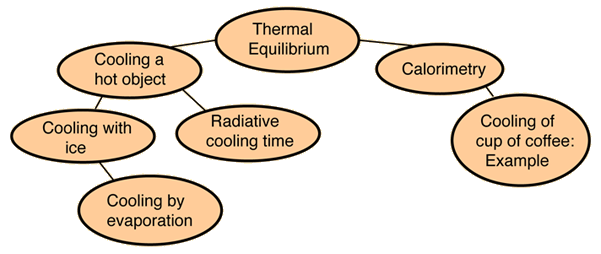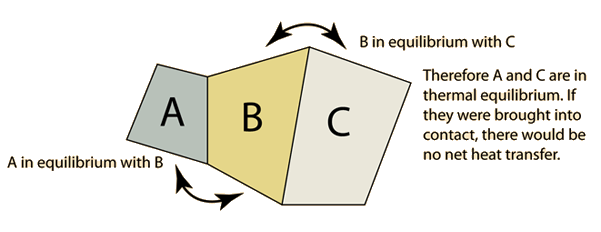# Thermal Equilibrium

It is observed that a higher temperature object which is in contact with a lower temperature object will transfer heat to the lower temperature object. The objects will approach the same temperature, and in the absence of loss to other objects, they will then maintain a constant temperature. They are then said to be in thermal equilibrium. Thermal equilibrium is the subject of the Zeroth Law of Thermodynamics.Index

Heat transfer concepts

 HyperPhysics***** Thermodynamics R Nave
Go Back

# Zeroth Law of Thermodynamics

The "zeroth law" states that if two systems are at the same time in thermal equilibrium with a third system, they are in thermal equilibrium with each other.If A and C are in thermal equilibrium with B, then A is in thermal equilibrium with C. Practically this means that all three are at the same temperature, and it forms the basis for comparison of temperatures. It is so named because it logically precedes the First and Second Laws of Thermodynamics.

There are underlying ideas about heat associated with the zeroth law of thermodynamics, and one of those ideas was expressed by Maxwell as "All heat is of the same kind." If A is in thermal equilibrium with B, then every unit of internal energy that passes from A to B is balanced by the same amount of energy passing from B to A. This is true even if the atomic masses in A are different from those in B, and even if the amount of energy per unit mass in A is different because the material has a different specific heat. This implies that there is a measurable property that can be considered to be the same for A and B, a property upon which heat transfer depends. That property is called temperature.

Index

Heat transfer concepts

 HyperPhysics***** Thermodynamics R Nave
Go Back